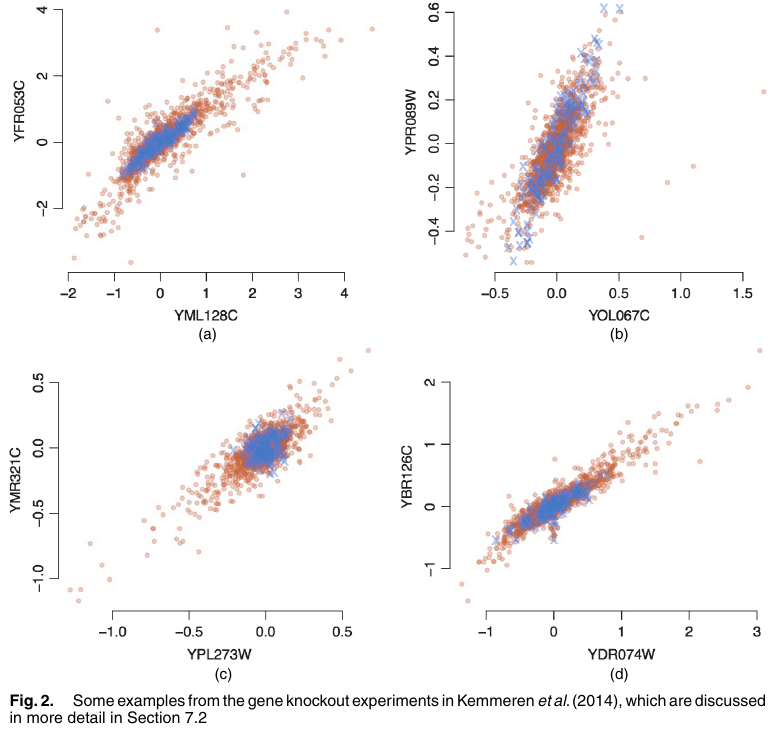# Causal Inference by Invariant Prediction

##### Posted on Nov 19, 20210 Comments

Causal inference by using invariant prediction: identification and confidence intervals

A primary goal in many applications: infer cause-effect relationships between variables

• the framework of potential outcomes and counterfactuals
• structural equation modelling
• graphical modelling

In the context of unknown casual structure, a typical approach for casual discovery is to

• characterize the Markov equivalence class of structures (or graphs)
• estimate the correct Markov equivalence class on the basis of observational or interventional data
• infer the identifiable casual effects or to provide some bounds

Within the framework of structural equation models (SEMs), work for fully identifiable structures exploiting additional restrictions such as

• non-Gaussianity
• non-linearity
• equal error variances

The paper propose a new method for casual discovery.

• if we consider all “direct causes” of a target variable of interest, then the conditional distribution of the target given the direct causes will not change when we interfere experimentally with all other variables in the model except the target itself.
• whereas it is well known that casual models have an invariance property, the paper try to exploit this fact for inference
• the proposed procedure gathers all submodels that are statistically invariant across environments in a suitable sense. The causal submodel consisting of the set of variables with a direct causal effect on the target variable will be one of these invariant submodels, with controlled high probability, and this allows us to control the probability of making false causal discoveries.

The method is tailored for the setting where data from different experimental settings or regimes, such as

• two different interventional data samples
• a combination of observational and interventional data

The method does not require knowledge of the location of interventions,

## Data from multiple environments or experimental settings

• different experimental conditions $e\in\cE$
• iid sample of $(X^e, Y^e)$ in each environment
• $X^e\in\IR^p$ is a predictor variable
• $Y^e\in\IR$ is a target variable of interest.

If a subset $S^\star\subset {1,\ldots,p}$ is causal for the prediction of a response $Y$, we assume that

• for all $e\in\cE$, $X^e$ has an arbitrary distribution and
• $Y^e=g(X^e_{S^\star}, \varepsilon^e),$ where $\varepsilon^e\sim F_\varepsilon$ and $\varepsilon^e\ind X_{S^\star}^e$.

where $g$ are assumed to be the same for all the experimental settings.

It reminds me of the common principal components.

## Assumed invariance of causal prediction

There is a vector of coefficients $\gamma^\star = (\gamma_1^\star,\ldots,\gamma_p^\star)^T$ with support $S^\star = {k:\gamma_k^\star\neq 0}\subset{1,\ldots,p}$ that satisfies

• for all $e\in\cE$, $X^e$ has an arbitrary distribution and
• $Y^e=\mu + X^e\gamma^\star + \varepsilon^e$, where $\varepsilon^e\sim F_\varepsilon$ and $\varepsilon^e\ind X_{S^\star}^e$Consider a linear SEM for the variables $(X_1=Y, X_2,\ldots, X_p, X_{p+1})$, with coefficients $(\beta_{jk})_{j,k=1,\ldots,p+1}$, whose structure is given by a directed acyclic graph. The independence assumption on the noise variables can here be replaced by the strictly weaker assumption that $\varepsilon_1^e\ind{\varepsilon_j^e;j\in AN(1)}$ for all environments $e\in\cE$, where $AN(1)$ are the ancestors of $Y$. Then assumptions 1 holds for the parents of $Y$, namely $S^\star = PA(1)$, and $\gamma^\star = \beta_1$, under the following assumption:

for each $e\in\cE$, the experimental setting $e$ arises by one or several interventions on variables from ${X_2,\ldots,X_{p+1}}$ but interventions on $Y$ are not allowed; here both do and soft interventions are allowed.

### Plausible causal predictors and identifiable causal predictors

Define

$H_{0,\gamma, S}(\cE): \gamma_k=0 \text{ if } k\not\in S \text{ and ...}$
• plausible causal predictors: the variables $S$ under $\cE$ if $H_{0,S}(\cE)$ is true.
• identifiable causal predictors: subset of plausible causal predictors
$S(\cE) = \cap_{S:H_{0,S}(\cE) \text{is true}} S$

### Plausible causal coefficients

• plausible causal coefficients: $\Gamma_S(\cE)={\gamma\in\IR^p: H_{0,\gamma, S}(\cE) \text{ is true}}$

the set of plausible causal coefficients for a set $S$ is either empty or contains only the population regression vector

Published in categories Note